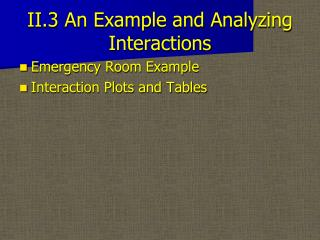DownloadDownload PresentationII.3 An Example and Analyzing Interactions

# II.3 An Example and Analyzing Interactions

Télécharger la présentation## II.3 An Example and Analyzing Interactions

- - - - - - - - - - - - - - - - - - - - - - - - - - - E N D - - - - - - - - - - - - - - - - - - - - - - - - - - -
##### Presentation Transcript

1. II.3 An Example and Analyzing Interactions • Emergency Room Example • Interaction Plots and Tables

2. Emergency Room Example • Quality of Care Index (10 to 50) • Design factors: • FactorLevels (Lo,Hi)A: Lab Coat White, Green B: Patient Gender Female, Male C: Caregiver Gender Male, Female • The 8 Standard Runs of the 23 Design Were Randomly Ordered, and Each Combination of Factors was observed

3. Emergency Room ExampleReport Form

4. Emergency Room ExampleStandard Order

5. Emergency Room ExampleCube Plot

6. Emergency Room ExampleEstimated Effects

7. Emergency Room ExampleEffects Probability Plot

8. Emergency Room Example • What do you see in the effects probability plot? • The Presence of a Strong Interaction Causes Some Interpretation Problems - the Interacting Factors B and C Must be Discussed as a "Team".

9. Interaction Plots and TablesInteraction Plots • A Tool for Aiding Interpretation of Two-Way Interactions.

10. Interaction Plots and TablesInteraction PlotsConstruction • 1. For a Given Pair of Factors Find the Average Response at Each of Their Four Level Combinations. • 2. Plot These with Response on the Vertical Axis, Using One of the Factor’s Levels on the Horizontal Axis. Connect and Label the Averages with the Same Level of the Other Factor.

11. Interaction Plots and TablesInteraction PlotsInterpretation • 1. If the Lines are Roughly Parallel, There is No Strong Interaction. • 2. If There is Interaction, the Plot Shows Clearly the Effect of a Factor at Each of the Levels of the Other Factor. • 3. Maximizing and Minimizing Combinations of the Factors are Easily Identified on the Plot and in the Table.

12. Emergency Room ExamplePatient*Caregiver Interaction Table • Let’s Construct an Patient*Caregiver Interaction Table for the Emergency Room Data

13. Emergency Room ExamplePatient*Caregiver Interaction Plot

14. Emergency Room ExerciseU-Do-It AC Interaction Table and Plot • Construct an AC interaction Table and Plot for the Emergency Room Data. • (Note in Practice We Would Not Do an AC Interaction Analysis for This Data Because the Estimate AC is Small - We Do It Here for Comparison Purposes and Practice).

15. InteractionsMore on Expected Responses • When there is Strong Interaction, we need a rule to compute estimated responses. Suppose we want to know the best expected response for Male Patients • We would want Male Doctors (C=-1) to administer to the Male Patients (B=1) in White Lab Coats (A=-1) • The estimated response should be computed as:

16. Interactions • In general, we start with a set of signs for the factors, • Multiply each important effect by its sign. We find the correct sign of interaction effects by multiplying together the signs of the main effects that comprise the interaction • Add all the signed effects then divide by 2 • Add to the overall mean • U-do-it: find the optimal estimated response for a female patient

17. Emergency Room ExerciseResults of the Experiment • The treatment factor under study, lab coat design, had an effect • An even stronger effect, the gender preference interaction, was discovered

18. Emergency Room ExerciseResults of the Experiment • A recoding of the factors can result in a simpler presentation—replace C with C*, Gender Match (Lo=N, Hi=Y). • An interaction in the original factors may simply be a main effects model in the fundamentalfactors.

19. Emergency Room ExampleRecoding Factors

20. Emergency Room ExampleCube Plot for Recoded Factors

21. Emergency Room ExampleEffects Plot for Recoded Factors